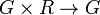# Group powered over a unital ring

## Definition

Suppose$G$ is a group and$R$ is a unital ring. A structure of$G$ as a group powered over$R$ includes an operation$G \times R \to G$ denoted by exponentiation, i.e., the output of$(g,r)$ is denoted$g^r$, satisfying the following conditions:

• For$r$ an integer (modulo whatever is the characteristic of the ring),$g^r$ is the usual$g^r$.
• For$r,s \in R$,$(g^r)^s = g^{rs}$.
• For$r,s \in R$,$(g^r)(g^s) = g^{r + s}$

## As a variety of algebras

For any fixed unital ring$R$, the groups powered over$R$ form a variety of algebras. This variety admits the variety of groups as a reduct, i.e., every group powered over a unital ring gives rise to a group.

## Functors

Suppose$R$ and$S$ are unital rings and$\varphi:R \to S$ is a homomorphism of unital rings. Then, if$G$ is a group equipped with a powering structure over$S$, we naturally get a powering structure of$G$ over$R$.

## Particular cases

### Cases based on rings

Value of ring Notion of group powered over that ring Is the powering uniquely determined by the abstract group structure? Does every group admit a powering over such a ring? Note that this condition would hold if the ring has a surjective ring homomorphism to$\mathbb{Z}$.$\mathbb{Z}$ usual concept of group Yes Yes$\mathbb{Z}[\pi^{-1}]$ where$\pi$ is a set of primes group powered over a set of primes where the set of primes is$\pi$. In other words, every element of the group has a unique$p^{th}$ root in the group. Yes No (unless$\pi$ is empty)$\mathbb{Q}$ rationally powered group: unique$p^{th}$ roots for all primes$p$ Yes No$\mathbb{Z}/n\mathbb{Z}$ for a positive integer$n$ group whose exponent is finite and divides$n$ Yes No$\mathbb{Z}[t]$ group along with a set map from the group to itself (need not be a homomorphism) that commutes with powering and sends every element to an element that it commutes with. No Yes$\mathbb{Q}[t]$ rationally powered group along with a set map from the group to itself that commutes with powering and sends every element to an element that commutes with it. No No